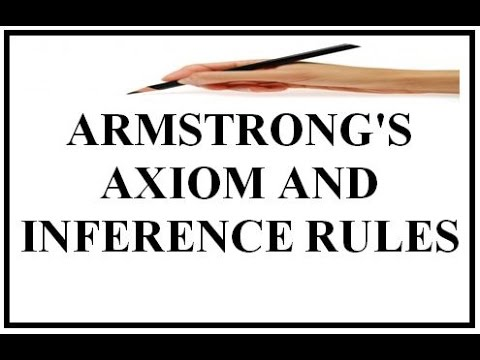The concept of functional dependence appears in several contexts of mathematics and logic and refers to certain mathematical entities can be expressed as mathematical functions of other entities. The concept of functional dependence is a generalization of the concept of linear dependence. It is said that a set of functions is functionally dependent when there is a functional relationship between them, or alternatively, when some of the functions of the set is expressible as a function of the other functions of the set.

### Functional DependenceA functional dependency, denoted by X -> Y, between two sets of attributes X and Y that are subsets of R (R = {A1, A2, …, A3}) specifies a restriction on the possible tuples that could form an example of relation r of R. The restriction says that, for any two tuples t1 and t2 of r such that t1 [X] = t2 [X], we must also have t1 [Y] = t2 [Y]. This means that the component Y values ​​of a tuple of r depend on or are determined by the values ​​of component X; or else, that the values ​​of the X component of a tuple determine in a unique way (or functionally) the values ​​of the component Y. We also say that there is a functional dependence from X to Y or that Y depends functionally on X.

Let a and b be attributes of the same table or relation T. It is said that b is functionally dependent on a and is denoted Ta -> Tb or simply a -> b if every possible value of a has a unique value of b, or what is associated with it. it is the same, in all the tuples of T in which the attribute a takes the same value v1, the attribute b also takes the same value v2. Clearly a -> b does not imply b -> a. The values ​​of attribute b can be repeated for different values ​​of a. A single attribute can functionally determine several attributes which is denoted by -> (b1, b2, …).

There can be a mutual functional dependence: a -> b and b -> a or what is the same to <-> b. Note that the concept of functional dependence does not depend on the concrete extension (content) that at any given moment the table has, but on any possible extension it might have.

The attributes a and b can be simple or compound (formed by the aggregation of several attributes). The functionally dependent attributes may or may not be part of the primary key of the table, of an alternative key or of a foreign key of another table.

The attribute b is functionally completely dependent on a if a -> b and b does not functionally depend on any subset of attributes of a. If a is a simple attribute and a -> b then the functional dependency is with complete security.

To Get info on Functional Testing visit the link.

### The Armstrong axiomsThe functional dependencies verify a series of properties called Armstrong axioms:

• Reflexivity: He can always deduce himself from any attribute or set of attributes. Trivial dependence: x -> x.
• Increase: If x -> and then x + z -> y. Thus the antecedent of a dependency can be trivially increased. Example: if the name of a person is determined with the ID, then with the ID plus the address, the name is also determined.
• Projectivity: If x -> y + z then x -> y. Example: if from the ID it is possible to deduce the name and address of a person, then with the ID it is possible to determine the name.
• Additivity: If x -> y and z -> w then x + z -> y + w. Example: if the name and address of a person’s telephone is determined with the IDN, then the name and telephone number can be determined with the ID and address.
• Transitivity or functional dependencies link: If x -> and e y -> z then x -> z. Example: if the code of the province of residence of a person can be determined with the IDN and with this code the name of the province can be determined, then with the IDN the nameo of the province can be determined. This is the basic mechanism of operation of the link between tables from foreign keys.